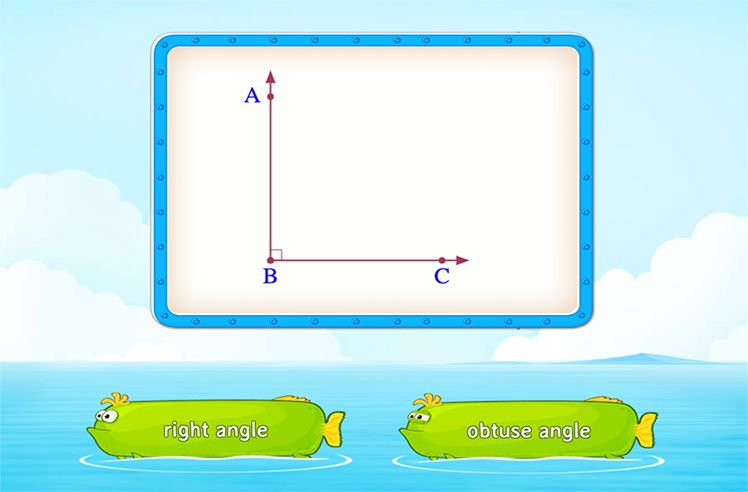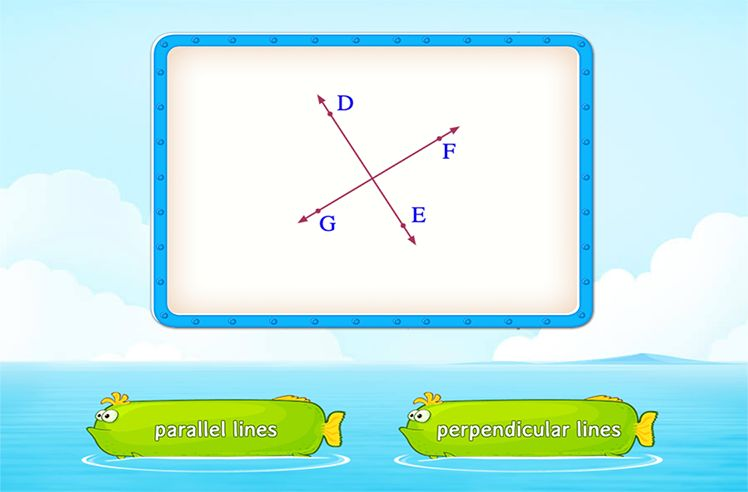# Angle Measure – Definition with Examples

» Angle Measure – Definition with Examples

## What is an Angle Measure?

In geometry, an angle measure can be defined as the measure of the angle formed by the two rays or arms at a common vertex.

Angles are measured in degrees ( °), using a protractor

##Let’s sing!

An acute angle is a little so small,
Right angle in the corner of a wall,
Obtuse angle at 2:50 in day-time,
Straight angle in a straw in soda-lime!
So many angles all around, even in a mime!

##Let’s do it!

Model and show how to use a protractor correctly. Ask your child to then use the protractor to measure and identify angles, basis their measurement.Shapes

Play NowAngles

Play NowParallel and Perpendicular Lines

Play Now